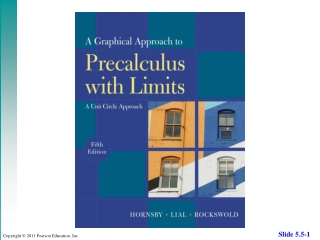DownloadDownload PresentationChapter 5: Exponential and Logarithmic Functions

# Chapter 5: Exponential and Logarithmic Functions

Download Presentation## Chapter 5: Exponential and Logarithmic Functions

- - - - - - - - - - - - - - - - - - - - - - - - - - - E N D - - - - - - - - - - - - - - - - - - - - - - - - - - -
##### Presentation Transcript

1. Chapter 5: Exponential and Logarithmic Functions 5.1 Inverse Functions 5.2 Exponential Functions 5.3 Logarithms and Their Properties 5.4 Logarithmic Functions 5.5 Exponential and Logarithmic Equations and Inequalities 5.6 Further Applications and Modeling with Exponential and Logarithmic Functions

2. 5.5 Exponential and Logarithmic Equations and Inequalities • Properties of Logarithmic and Exponential Functions • For b > 0 and b  1, • if and only if x = y. • If x > 0 and y > 0, then logb x = logb y if and only if x = y.

3. 5.5 Exponential and Logarithmic Equations and Inequalities • Type I Exponential Equations • Solved in Section 5.2 • Easily written as powers of same base i.e. 125x = 5x • Type 2 Exponential Equations • Cannot be easily written as powers of same base i.e 7x = 12 • General strategy: take the logarithm of both sides and apply the power rule to eliminate variable exponents.

4. 5.5 Type 2 Exponential Equations Example Solve 7x = 12. Solution

5. 5.5 Solving a Type 2 Exponential Inequality Example Solve 7x < 12. Solution From the previous example, 7x = 12 when x 1.277. Using the graph below, y1 = 7xis below the graph y2 = 12 for all x-values less than 1.277. The solution set is (–,1.277).

6. 5.5 Solving a Type 2 Exponential Equation Example Solve Solution Take logarithms of both sides. Apply the power rule. Distribute. Get all x-terms on one side. Factor out x and solve.

7. 5.5 Solving a Logarithmic Equation of the Type log x = log y Example Solve Analytic Solution The domain must satisfy x + 6 > 0, x + 2 > 0, and x > 0. The intersection of these is (0,). Quotient property of logarithms log x = log y x = y

8. 5.5 Solving a Logarithmic Equation of the Type log x = log y Multiply by x + 2. Since the domain of the original equation was (0,), x = –3 cannot be a solution. The solution set is {2}. Solve the quadratic equation.

9. 5.5 Solving a Logarithmic Equation of the Type log x = log y Graphing Calculator Solution The point of intersection is at x = 2. Notice that the graphs do not intersect at x = –3, thus supporting our conclusion that –3 is an extraneous solution.

10. 5.5 Solving a Logarithmic Equation of the Type log x = k Example Solve Solution Since it is not in the domain and must be discarded, giving the solution set Write in exponential form.

11. 5.5 Solving Equations Involving both Exponentials and Logarithms Example Solve Solution The domain is (0,). –4 is not valid since –4 < 0, and x > 0.

12. 5.5 Solving Exponential and Logarithmic Equations • An exponential or logarithmic equation can be solved • by changing the equation into one of the following • forms, where a and b are real numbers, a > 0, and a 1: • a f(x) = b • Solve by taking the logarithm of each side. • loga f(x) = loga g(x) • Solve f(x) = g(x) analytically. • 3. loga f(x) = b • Solve by changing to exponential form f(x) = ab.

13. 5.5 Solving a Logarithmic Formula from Biology Example The formula gives the number of species in a sample, where n is the number of individuals in the sample, and a is a constant indicating diversity. Solve for n. Solution Isolate the logarithm and change to exponential form.